Function Repository Resource:

# MeanCurvature

Compute the mean curvature of a surface

Contributed by: Wolfram Staff (original content by Alfred Gray)
 ResourceFunction["MeanCurvature"][s,{u,v}] computes the mean curvature of parametrized surface s with respect to parametrizing variables u and v. ResourceFunction["MeanCurvature"][eq,{x,y,z}] computes the mean curvature of the surface given by the implicit equation eq in variables x,y and z.

## Details

Mean curvature can be defined as the mean of the principal curvatures, while Gaussian curvature is the product of principal curvatures.
Mean curvature is an extrinsic measure of a surface and locally describes the curvature of an embedded surface.
Mean curvature can be derived as well from the first and second fundamental forms.

## Examples

### Basic Examples (1)

Mean curvature of a sphere:

 In:=Out=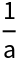### Scope (2)

Plot the Kuen surface:

 In:=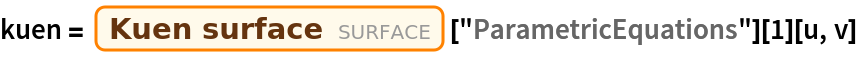Out=In:=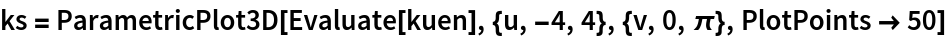Out=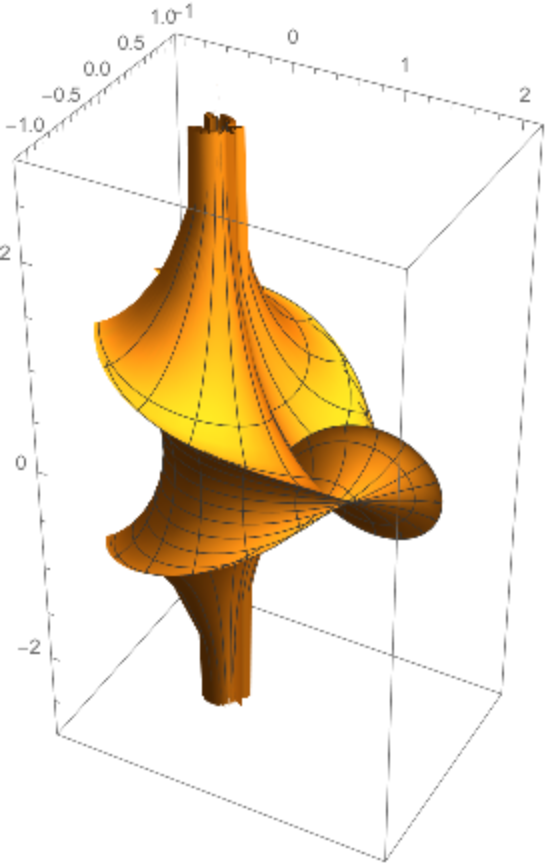Compute its mean curvature:

 In:=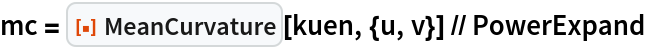Out=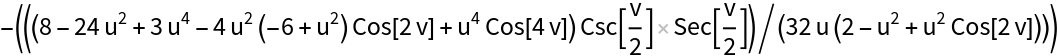Plot the mean curvature:

 In:=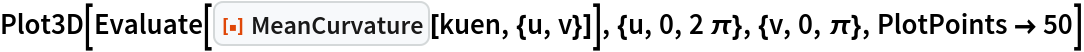Out=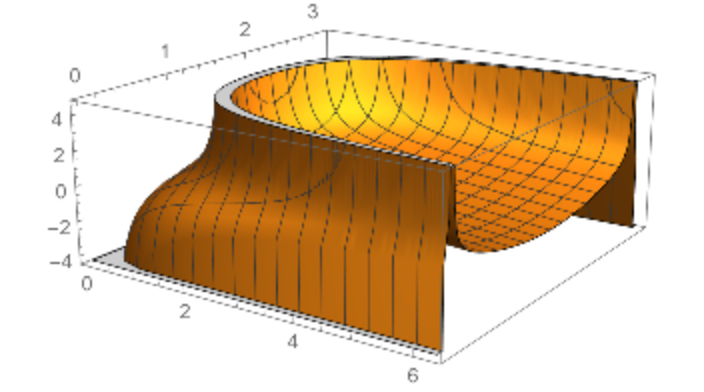Plot the surface with a color function in accordance with the mean curvature:

 In:=Out=The Gaussian curvature is constant:

 In:=Out=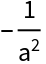Define the implicit equation for the sine surface:

 In:=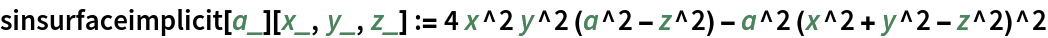The mean curvature:

 In:=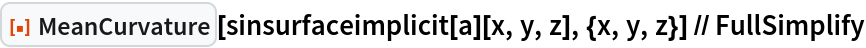Out=In:=Out=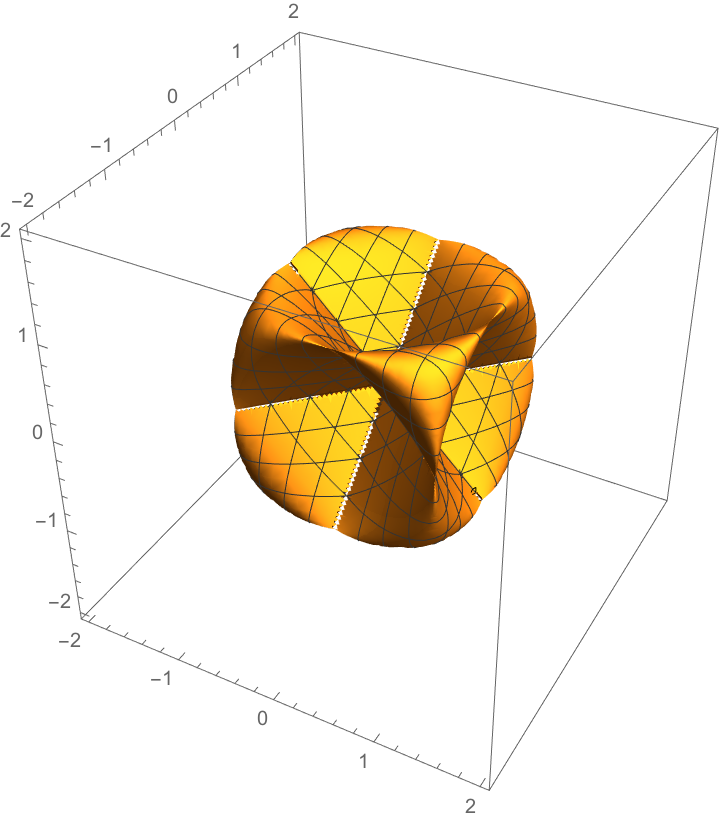### Properties and Relations (3)

The mean curvature of a minimal surface is zero:

 In:=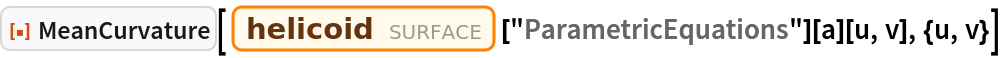Out=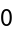The mean curvature for an implicit surface:

 In:=Out=The curvature can be obtained for named surfaces using entities:

 In:=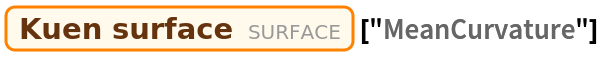Out=The expressions seem to be different, but they are equal:

 In:=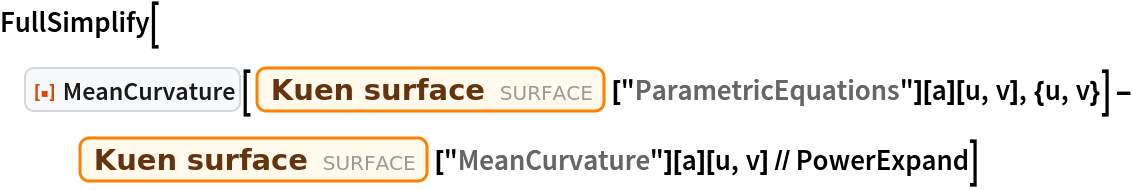Out=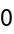Enrique Zeleny

## Version History

• 1.1.0 – 19 July 2021
• 1.0.0 – 11 March 2020